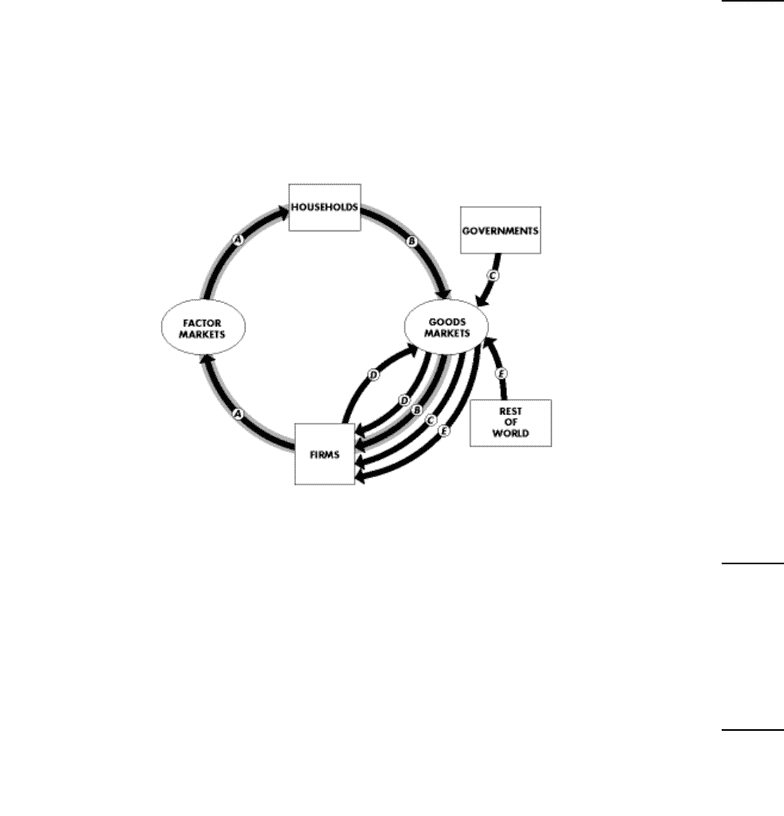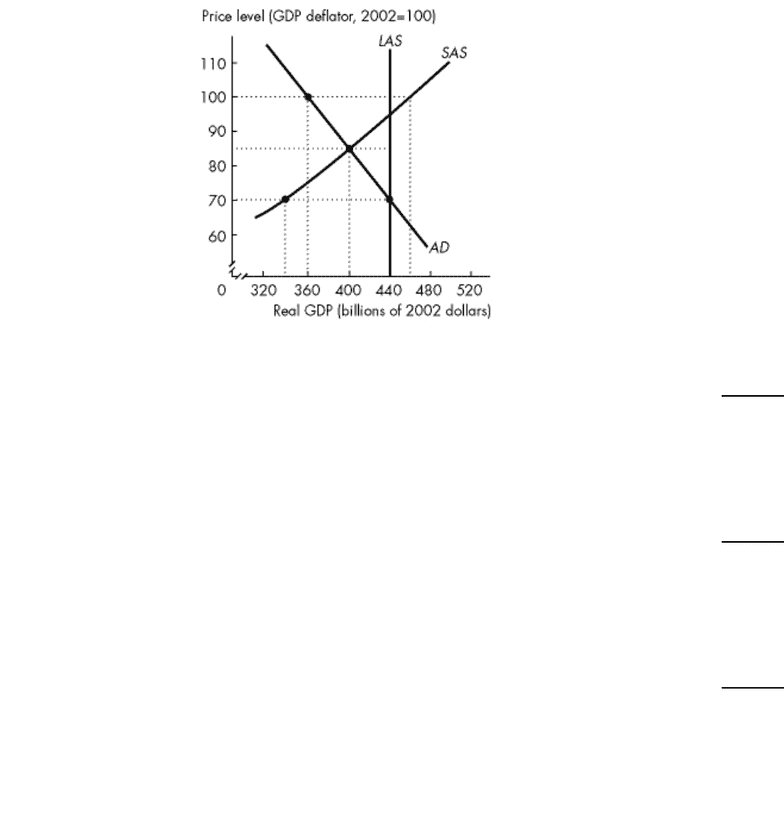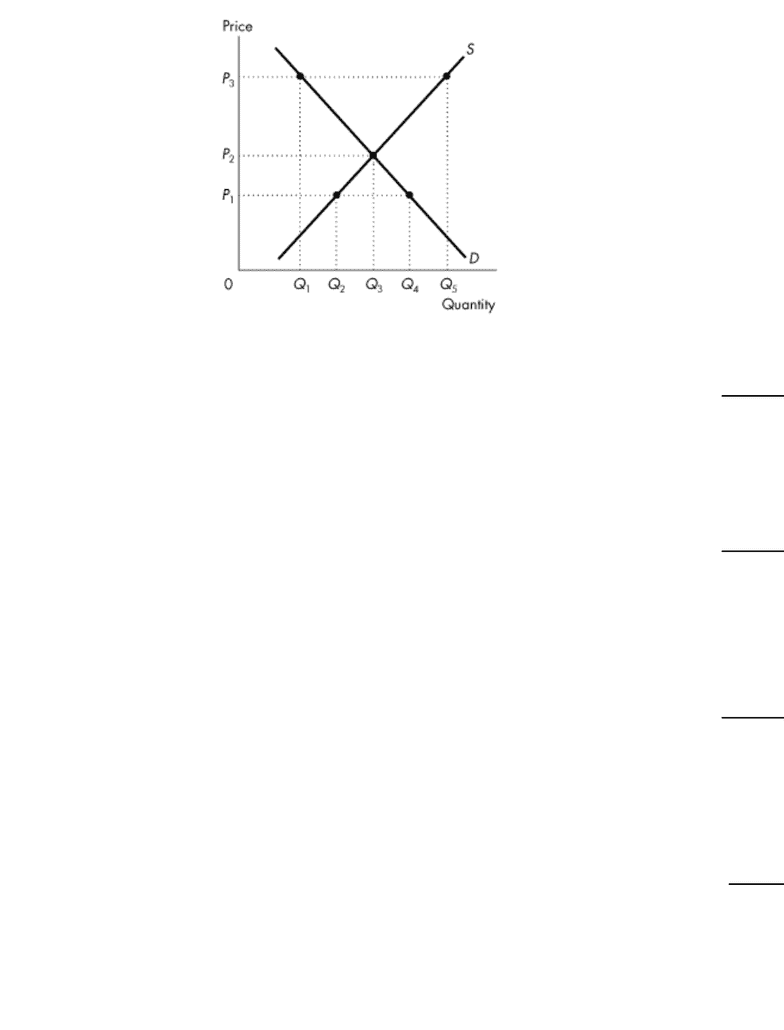Study Guides (390,000)
CA (150,000)
York (10,000)
ECON (900)
ECON 1010 (200)
All (70)
Midterm

# ECON 1010 Study Guide - Midterm Guide: Consumption Function, Monetarism, UnemploymentExam

Department
Economics
Course Code
ECON 1010
Professor
All
Study Guide
Midterm

This preview shows pages 1-3. to view the full 17 pages of the document.Exam
Name___________________________________
Macro Economics 1010.N Mid Term Test Winter 2012---TEST C
MULTIPLE CHOICE. Choose the one alternative that best completes the statement or answers the question.
1)
Suppose there is an increase in the quantity of capital. As a result, the SAS
1)
A)
shifts rightward, but the LAS curve does not shift.
B)
and the LAS curves both shift leftward.
C)
curve does not shift but the LAS curve shifts rightward.
D)
curve does not shift but the LAS curve shifts leftward.
E)
and the LAS curves both shift rightward.
Use the figure below to answer the following questions.
Figure 20.1.1
2)
Refer to Figure 20.1.1, which shows the circular flow of expenditure and income for Venus. During
2008, A was \$100, B was \$50, C was \$30 and D was \$10. How much is aggregate expenditure?
2)
A)
\$50.
B)
\$75.
C)
\$90.
D)
\$100.
E)
None of the above.
3)
The fixed basket of Econoland consists of 10 units of A, 20 units of B, and 30 units of C. Current
prices are \$1 per unit of A, \$2 per unit of B, and \$3 per unit of C. Base year prices are \$1 for each
unit of A,B, and C. What is the CPI in the current year?
3)
A)
140
B)
233
C)
43
D)
430
E)
100
1

Only pages 1-3 are available for preview. Some parts have been intentionally blurred.Use the figure below to answer the following questions.
Figure 26.3.1
4)
Refer to Figure 26.3.1. Short-run macroeconomic equilibrium real GDP in Econoworld is
4)
A)
\$360 billion.
B)
\$480 billion.
C)
\$400 billion.
D)
\$440 billion.
E)
\$520 billion.
5)
Which one of the following is false?
5)
A)
Y+M=C+I+ G + X
B)
Y=C+I+G+M-X
C)
Y=C+I+G+X- M
D)
Y- C - I - G - X + M = 0
E)
X- M = Y - C - I - G
6)
The saving function shows the relationship between saving and
6)
A)
disposable income.
B)
the price level.
C)
nominal income.
D)
the interest rate.
E)
consumption.
2

Only pages 1-3 are available for preview. Some parts have been intentionally blurred.Use the figure below to answer the following questions.
Figure 3.4.1
7)
At price P3 in Figure 3.4.1,
7)
A)
there is a surplus in the amount of Q5-Q1.
B)
there is a shortage in the amount of Q5-Q1.
C)
equilibrium quantity is Q5.
D)
this market is in equilibrium.
E)
there is a tendency for the price to rise.
8)
Without money to act as a medium of exchange,
8)
A)
independence in production would lead to a proliferation of new products.
B)
barter exchange would allow for a much simpler yet increased standard of living.
C)
the standard of living in the economy would increase.
D)
the increased transaction costs associated with trading would prohibit some trades from
taking place.
E)
all exchanges that take place under a monetary system would still take place.
9)
Everything else remaining the same, which one of the following would increase equilibrium real
GDP?
9)
A)
a decrease in exports
B)
an increase in exports
C)
a decrease in investment
D)
an increase in saving
E)
an increase in taxes
10)
If the labor force participation rate is rising and the working-age population is not changing, then
the
10)
A)
number of employed people must be increasing.
B)
number of unemployed people is falling and the size of the labor force is rising.
C)
number of unemployed people is rising and the size of the labor force is falling.
D)
size of the labor force is falling.
E)
size of the labor force is rising.
3